# Geometry – fundamental statements

A point is symbolized by a dot and represents a specific location. It has no sixe nor shape.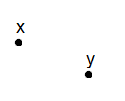A line is defined as a line of points that extends infinitely in two directions which is shown by the arrowheads. A line is defined by two points on the line and has only one dimension.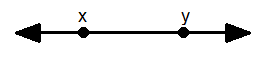A line segment is a part of a line that has two defined endpoints. A line segment represents a collection of points inside the endpoints and it is named by its endpoints.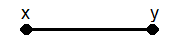A ray is a line that only has one defined endpoint and one side that extends endlessly away from the endpoint. A ray is named by its endpoint and by another point on the line.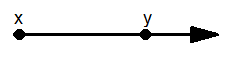The angle that is formed by two rays that have the same endpoint is called the vertex. The vertex is measured in degrees and is easiest measured by using a protractor.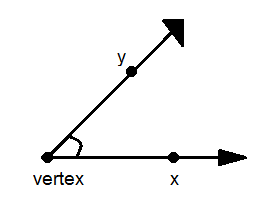You can measure angles by using a protractor.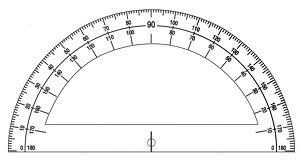Angles can be classified according to how big they are. A right angle has a measure of 90° whereas an angle that measures between 0° and 90° is called an acute angle. An angle that measures between 90° and 180° is called an obtuse angle whereas a straight angle measures 180°.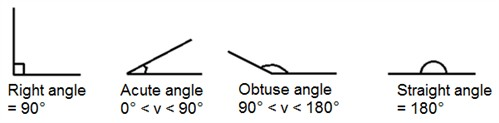A flat surface without edges and boundaries is called a plane. It extends infinitely in two dimensions and is named by three points in the plane that are not on the same line e.g. xyz. An example of a plane would be the coordinate plane.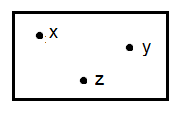Two lines that meet in a point are called intersecting lines

Two lines, that are in the same plane and that will never intersect are called parallel lines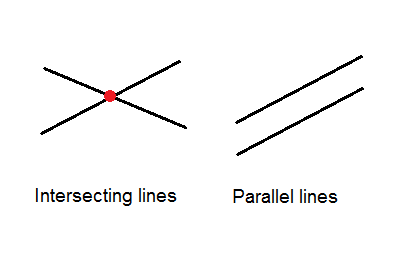Lines (or rays) that are perpendicular are lines that intersect in a right angle. The symbol for perpendicular is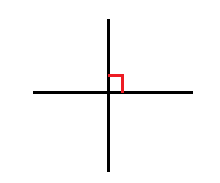Skew lines are lines that are not in the same plane.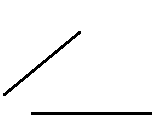## Video lesson

Classify all angles